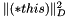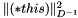ConicBundle
ConicBundle::MinorantPointer Class Reference

points to MinorantUseData that may be shared by many and allows computations with Minorants More...

`#include <MinorantPointer.hxx>`

Inheritance diagram for ConicBundle::MinorantPointer:## Public Member Functions

MinorantPointeroperator= (const MinorantPointer &mp)
calls new_data with the data of mp and copies the modification_id

void init (const MinorantPointer &mp, CH_Matrix_Classes::Real factor=1., bool enforce_copy=false)
if factor!=1 it generates another MinorantUseData referring to the one of mp, otherwise it simply uses the same one (empty stays empty)

void init (Minorant *mp, CH_Matrix_Classes::Integer modification_id=-1, CH_Matrix_Classes::Real factor=1.)
if mp==0 it becomes empty, otherwise it creates and then points to a MinorantUseData for holding mp with this modification_id and factor

MinorantPointer ()
declares the pointer empty

MinorantPointer (MinorantUseData *in_md)
initialize this to point to in_md

MinorantPointer (const MinorantPointer &mp)
calls new_data

MinorantPointer (const MinorantPointer &mp, CH_Matrix_Classes::Real factor)
calls init(const MinorantPointer&,CH_Matrix_Classes::Real)

MinorantPointer (Minorant *mnrt, CH_Matrix_Classes::Integer modification_id, CH_Matrix_Classes::Real factor=1.)
calls init(Minorant*,CH_Matrix_Classes::Integer,CH_Matrix_Classes::Real)

virtual ~MinorantPointer ()
calls delete_data

void clear ()
afterwards the pointer is empty (calls delete_data())

int synchronize_ids (CH_Matrix_Classes::Integer new_modification_id, CH_Matrix_Classes::Integer new_center_id, CH_Matrix_Classes::Integer old_center_id, CH_Matrix_Classes::Integer new_cand_id, CH_Matrix_Classes::Integer old_cand_id, CH_Matrix_Classes::Integer new_prex_id=0)
if not empty it sets the modification_id to its new id and reinitializes the evaluation map

bool empty () const
returns true if the pointer is empty

bool valid () const
returns true if the pointer is not empty and the data is valid

bool zero () const
returns true if the pointer is not empty but all entrys (also the offset) are zero

bool aggregate () const
returns ture if it points to a combination of minorants from the same function; More...

bool one_user () const
returns true if not valid or this is the only active pointer to the minorant

const Minorantget_minorant () const
returns the Minorant *this points to or 0 if empty

int get_scaleval_and_minorant (CH_Matrix_Classes::Real &sv, Minorant *&m)
returns the Minorant *this points to with its scaling value or 0 if empty

const PrimalDataget_primal ()
returns the primal of the Minorant *this points to or 0 if empty (first carrying out any pending scalings on the minorant)

CH_Matrix_Classes::Real offset () const
returns the offset of the minorant (including the internal scalings) or CB_minus_infinity if empty

CH_Matrix_Classes::Real coeff (CH_Matrix_Classes::Integer i) const
returns coefficient i of the minorant (including the internal scalings) or 0. if empty

CH_Matrix_Classes::Integer nonzeros () const
returns the number of nonzero coefficients

int scale (CH_Matrix_Classes::Real val)
multiply the MinorantPointer by val (this is an external factor for the minorant and possibly its primal information, but it will be used in aggreagation and when retrieving the approximate primal)

int call_primal_extender (PrimalExtender &prex, CH_Matrix_Classes::Integer prex_id)
executes prex on the primal if its id is smaller then prex_id; returns != if this fails or not primal data is available

int apply_modification (const GroundsetModification &gsmdf, CH_Matrix_Classes::Integer mod_id, MinorantExtender *mex, bool apply_gsmdf_costs=false)
if valid and the local modification_id is smaller than mod_id, it modifies the coefficients as described by GroundsetModification; if successful, the mod_id is assigend and the function returns 0; otherwise it is invalidated and returns !=0; the costs of gsmdf are used only if apply_gsmdf_costs=false in whic case mex must be NULL

CH_Matrix_Classes::Real evaluate (CH_Matrix_Classes::Integer yid, const CH_Matrix_Classes::Matrix &y, bool with_constant=true) const
negative ids are allowed and indicate there is no need to memorize this result, returns CB_minus_infinity if empty, otherwise offset+ip(minorant,y)

add the offset; if empty, initialize to offset with zero linear part, if not the only user (use_cnt>1), clone it first

int get_minorant (CH_Matrix_Classes::Real &offset, CH_Matrix_Classes::Matrix &mat, CH_Matrix_Classes::Integer column, CH_Matrix_Classes::Real alpha=1., bool add=false, const CH_Matrix_Classes::Indexmatrix *skip_fixed=0, const CH_Matrix_Classes::Matrix *fixed_vals=0) const
store/add the minorant in offset and a matrix column, possibly skipping indices skip_fixed. The coordinats of the latter ones are multiplied by the given values or 0 and added to offset More...

int get_minorant (MinorantPointer &mp, CH_Matrix_Classes::Real alpha=1.) const
store/add the minorant in/to mp, possibly scaled by alpha More...

int get_minorant (MinorantPointer &mp, CH_Matrix_Classes::Real alpha, const CH_Matrix_Classes::Sparsemat *sp, const CH_Matrix_Classes::Indexmatrix *provided_row_indices=0, const CH_Matrix_Classes::Indexmatrix *needed_col_indices=0, bool enforce_copy=false) const
store/add the minorant in/to mp, possibly scaled by alpha and transformed by sp, which possibly requires only the indices of provided_row_indices to compute possibly only the indices in needed_col_indices More...

int aggregate (const MinorantBundle &minorants, const CH_Matrix_Classes::Matrix &coeff, CH_Matrix_Classes::Real factor=1.)
collect the aggregate as the nonnegative linear combination of the bundle minorants (here *this may not be part of the bundle!). If *this is not empty, add this aggregate to *this. Only aggregation carries along primal data! More...

int aggregate (const MinorantPointer &minorant, double itsfactor=1.)
aggregate the minorant*itsfactor to *this, both must be valid for this. Only aggregation carries along primal data!

CH_Matrix_Classes::Real ip (const MinorantPointer &mp, const CH_Matrix_Classes::Indexmatrix *skip_fixed=0, const CH_Matrix_Classes::Matrix *ipdiag=0) const
computes the inner product of the two minorants; if skip_fixed!=NULL the corrsponding indices are not considered, if ipdiag!=0 the inner product is taken with respect to this diagonal matrix, i.e. sum_i mp(i)*(this)(i)(*ipdiag)(i)

CH_Matrix_Classes::Real ip (const CH_Matrix_Classes::Matrix &m, const CH_Matrix_Classes::Matrix *ipdiag=0, CH_Matrix_Classes::Integer startindex_m=0) const
computes the inner product with m; if ipdiag!=0 the inner product is taken with respect to this diagonal matrix, i.e. sum_i mp(startindex_m+i)*(this)(i)(*ipdiag)(i)

bool operator< (const MinorantPointer &mp) const
they are equal if they point to the same object, compares the addresses of this objects

bool operator== (const MinorantPointer &mp) const
they are equal if they point to the same object, compares the addresses of this objects

bool operator> (const MinorantPointer &mp) const
they are equal if they point to the same object, compares the addresses of this objects

bool equals (const MinorantPointer &mp, CH_Matrix_Classes::Real tol=1e-10) const
they are equal if they point to the same object or are both 0. If not, they differ if their matrix representations differ; if not, they differ if the entries differ by at least tol*(1.+fabs(this->offset())

CH_Matrix_Classes::Real norm_squared (const CH_Matrix_Classes::Matrix *D=0) const
Compute the norm squared of this for the given diagonal matrix D (identity if not given), i.e..

CH_Matrix_Classes::Real dual_norm_squared (const CH_Matrix_Classes::Matrix *D=0) const
Compute the dual norm squared of this for the given diagonal matrix D (identity if not given), i.e..

CH_Matrix_Classes::Matrixleft_genmult (const CH_Matrix_Classes::Matrix &B, CH_Matrix_Classes::Matrix &C, CH_Matrix_Classes::Real alpha=1., CH_Matrix_Classes::Real beta=0., int thistrans=0, int btrans=0, CH_Matrix_Classes::Integer thisindex=0) const
computes and returns C=alpha*(*this)*B+beta*C where B and *this may be transposed and *this is considered to be a column with thisindex in a bigger matrix

CH_Matrix_Classes::Matrixright_genmult (const CH_Matrix_Classes::Matrix &A, CH_Matrix_Classes::Matrix &C, CH_Matrix_Classes::Real alpha=1., CH_Matrix_Classes::Real beta=0., int atrans=0, int thistrans=0, CH_Matrix_Classes::Integer thisindex=0) const
computes and returns C=alpha*A*(*this)+beta*C where A and *this may be transposed and *this is considered to be a column with thisindex in a bigger matrix

std::ostream & display (std::ostream &out, int precision=8) const
output the Minorant in a nice formatPublic Member Functions inherited from ConicBundle::CBout
virtual void set_out (std::ostream *out=0, int print_level=1)
Specifies the output level (out==NULL: no output at all, out!=NULL and level=0: errors and warnings, level>0 increasingly detailed information) More...

virtual void set_cbout (const CBout *cb, int incr=-1)
Specifies the output level relative to the given CBout class. More...

void clear_cbout ()
reset to default settings (out=0,print_level=1)

CBout (const CBout *cb=0, int incr=-1)
calls set_cbout

CBout (std::ostream *outp, int pl=1)
initialize correspondingly

CBout (const CBout &cb, int incr=0)
copy constructor

virtual bool cb_out (int pl=-1) const
Returns true if out!=0 and (pl<print_level), pl<0 should be used for WARNINGS and ERRORS only, pl==0 for usual output.

std::ostream & get_out () const
If cb_out() returned true, this returns the output stream, but it will abort if called with out==0.

std::ostream * get_out_ptr () const
returns the pointer to the output stream

int get_print_level () const
returns the print_level

virtual int mfile_data (std::ostream &out) const
writes problem data to the given outstream

## Private Member Functions

void delete_data ()
if -1 it is invalid or empty, otherwise it gives the modification id of the function that it was created for, at that time identical to the one in the MinorantUseData More...

void new_data (MinorantUseData *in_md)
first deletes the old data and then fills the information with the new

int prepare_for_changes (CH_Matrix_Classes::Real factor=1., bool with_primal=false)
check whether the minorant is owend by this pointer (use_cnt==1) and if not, clone it first

## Private Attributes

MinorantUseDatamd
if null, it is regarded as not initialized or empty

## Detailed Description

points to MinorantUseData that may be shared by many and allows computations with Minorants

A minorant pointer is empty if it does not point to any MinorantUseData

## ◆ aggregate() [1/2]

 bool ConicBundle::MinorantPointer::aggregate ( ) const

returns ture if it points to a combination of minorants from the same function;

A minorant is considered an aggregate if it is obtained from an aggregate or it was computed by a call to aggregate(MinorantBundle&,Real); It is not an aggregate if it is the sum of non aggregate minorants from different functions.

An empty pointer is not an aggregate.

Referenced by nonzeros(), and valid().

## ◆ aggregate() [2/2]

 int ConicBundle::MinorantPointer::aggregate ( const MinorantBundle & minorants, const CH_Matrix_Classes::Matrix & coeff, CH_Matrix_Classes::Real factor = `1.` )

collect the aggregate as the nonnegative linear combination of the bundle minorants (here *this may not be part of the bundle!). If *this is not empty, add this aggregate to *this. Only aggregation carries along primal data!

All minorants in the bundle need to be valid. Aggregating over no minorants is treated as a zero minorant without any primal information.

## ◆ delete_data()

 void ConicBundle::MinorantPointer::delete_data ( )
private

if -1 it is invalid or empty, otherwise it gives the modification id of the function that it was created for, at that time identical to the one in the MinorantUseData

reduces the use_cnt of the MinorantUseData it points to and deletes it if this reaches 0; afterwards it is empty

Referenced by clear(), and ~MinorantPointer().

## ◆ get_minorant() [1/3]

 int ConicBundle::MinorantPointer::get_minorant ( CH_Matrix_Classes::Real & offset, CH_Matrix_Classes::Matrix & mat, CH_Matrix_Classes::Integer column, CH_Matrix_Classes::Real alpha = `1.`, bool add = `false`, const CH_Matrix_Classes::Indexmatrix * skip_fixed = `0`, const CH_Matrix_Classes::Matrix * fixed_vals = `0` ) const

store/add the minorant in offset and a matrix column, possibly skipping indices skip_fixed. The coordinats of the latter ones are multiplied by the given values or 0 and added to offset

The dimensions of the matrix must already fit the requirements on input. If add is false (as by default), the full length of the column is initialized to the gradient and filled up with zeros where needed. skip_fixed and fixed_vals must both be given or both not be given. If they are both given, both must have the same length. If skip_fixed is given, it must have strictly increasing indices. The skip part of the routine is used in implementations of BundleScaling::get_QP_costs.

## ◆ get_minorant() [2/3]

 int ConicBundle::MinorantPointer::get_minorant ( MinorantPointer & mp, CH_Matrix_Classes::Real alpha = `1.` ) const

store/add the minorant in/to mp, possibly scaled by alpha

If mp is empty, store it there, if mp is not empty, add it.

if *this is empty, it causes an error.

## ◆ get_minorant() [3/3]

 int ConicBundle::MinorantPointer::get_minorant ( MinorantPointer & mp, CH_Matrix_Classes::Real alpha, const CH_Matrix_Classes::Sparsemat * sp, const CH_Matrix_Classes::Indexmatrix * provided_row_indices = `0`, const CH_Matrix_Classes::Indexmatrix * needed_col_indices = `0`, bool enforce_copy = `false` ) const

store/add the minorant in/to mp, possibly scaled by alpha and transformed by sp, which possibly requires only the indices of provided_row_indices to compute possibly only the indices in needed_col_indices

If mp is empty, store it there, if mp is not empty, add it.

if sp==0, it is treated as the identity

If provided_row_indices or needed_col_indices is given, its indices must be in strictly increasing order. For sp==0 both must coincide and will probably be ignored by just returning a scaled reference to *this in mp.

if *this is empty, it causes an error.

The documentation for this class was generated from the following file: